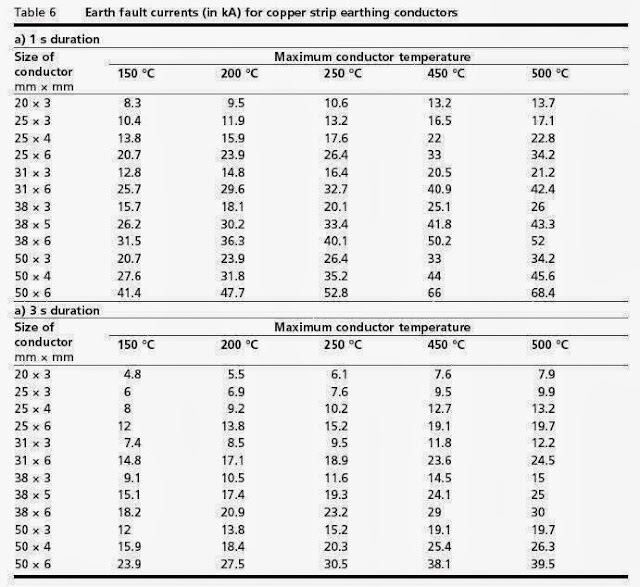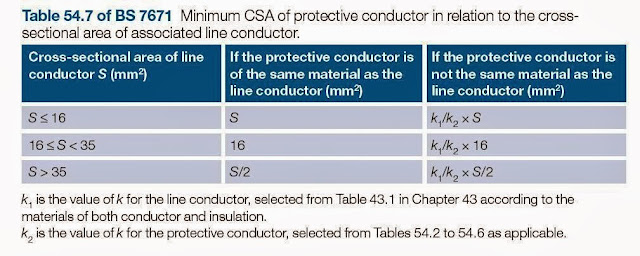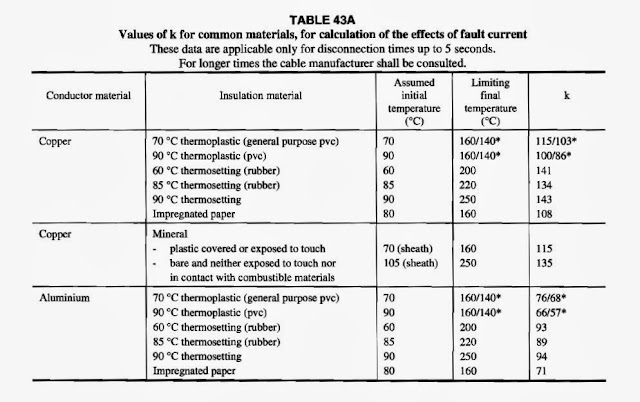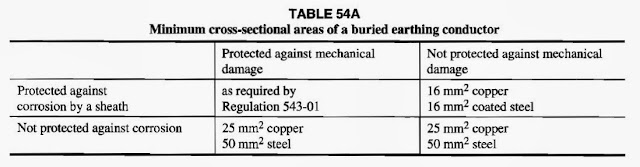# Grounding Design Calculations – Part Five

I indicated that the Earthing Systems Design Steps process has (3) main steps:

 Earthing Systems Design Steps A grounding system design process has (3) main steps: Data Collection, Data Analysis, Grounding Design Calculations.

And I explained the first step: Data Collection in the following Articles:

I explained the second step: Data Analysis in the following Articles:

And I explained What we are going to design for grounding system in any building in the following Articles:

And, in Article
Grounding Design Calculations – Part One ", I indicated the following:

 Grounding System Design Calculations according to type of the building The procedures for performing the Grounding System Design Calculations can differ slightly according to the type of the building as follows: Domestic, commercial and industrial premises, High and medium voltage electricity substations.

 First: Domestic, commercial and industrial premises We mean by domestic, commercial and industrial premises, all installations up to 1,000 V ac and 1,500 V dc - between phases, with some minor exceptions.

And I started discussion for Methods of Grounding Design Calculations of Domestic, commercial and industrial premises as follows:

 Methods of Grounding Design Calculations There are many methods can be used for performing Grounding System Design Calculations But the common methods are: Equations Method, Nomographs Method, Excel Spreadsheets Method, Tables Method, Online Earthing Calculators Method, Software Programs Method.

Grounding Design Calculations – Part Two ", I explained the first method of grounding design calculations: Equations Method and solved examples.

And I explained the second method of grounding design calculations: Nomographs Method in Article
" Grounding Design Calculations – Part Three".

Also, I explained third method of grounding design calculations: Excel Spreadsheets Method in Article " Grounding Design Calculations – Part Four ".

Today, I will explain the Other Methods for Performing of Grounding System Design Calculations.

 Third Method: Tables Method

 Important The Tables Method is used for sizing earthing conductors only, not for calculating the earth resistance value.

 Methods Of Sizing Earthing Conductors There are two methods for sizing protective conductors including earthing conductors as follows: By using the adiabatic equation, By using Tables.

 Method#1: By Using The Adiabatic Equation This method is used where: The choice of the Cross sectional area of the line conductors has been determined by consideration of short circuit current, or The earth fault current is expected to be less than the short circuit current. The cross-sectional area of the earthing conductors, where calculated, shall be not less than the value determined by the following equation: Where: S is the nominal cross-sectional area of the conductor in mm2. I is the average fault current, in A r.m.s. t is the fault current duration, in s. k is the r.m.s. current density, in A/mm2 . Notes: Application of the formula will in many instances result in a protective conductor having a smaller CSA than that of the live conductors of the associated circuit. This is quite acceptable. The cross-sectional area of the earthing conductors (s) are also calculated directly from our excel sheet for Excel Spreadsheet for Grounding System Design Calculations of Domestic, Commercial and Industrial Premises as shown in below image.(click the link to download your copy).

First: Getting K Value

To get values of K, we have (2) cases to be considered as follows:

Case#1: t= 1 s and initial conductor temperature of 30 °C

In this case, the value of K is given in below Table-1:Table-1

Case#2: t= 1 s and other initial/ final conductor temperature

In this case, the value of K is obtained from the following equation:

Where:

T1 is the initial temperature, in °C;
T2 is the final temperature, in °C;
K and β have the values given in Table-2.Table-2

Second: Getting The Average Fault Current Value (I)

To get values of average fault current (I), we have (2) methods:

• Method#1: by using Earth fault loop impedance,
• Method#2: from tables and equations.

Method#1: by using Earth fault loop impedance

The value of the fault current (I) will be determined from the following equation:

I = Uo / Zs

Where:

Uo the nominal Voltage
Zs the measured value of earth fault loop impedance corrected to the operating temperature of the conductor.

Getting value of Zs:

Zs = Ze + (R1 + R2)   ohm

Where:

Ze: the impedance of the supply side of the earth fault loop.( it can be obtained from utility companies but typical values are 0.35 ohm for TN-C-S supplies and 0.8 ohm for TN-S supplies),
R1: the resistance of the phase conductor,
R2: the resistance of the earth conductor.

Values of (R1 + R2) are given in the below table (table 9A of the IEE on site guide):Table 9A of the IEE on Site Guide

Note:

(R1 + R2) in above table is at 20◦C, for other temperatures, the value will be increased 2% for each 5◦C rise in temperature.

Example#1:

The design current for a circuit is 38A. The current carrying capacity of cable has been calculated and the circuit is to be wired in 70◦C thermoplastic singles, 6mm2 live conductors and 1.5mm2 circuit protective conductors, Supply is 230V TNS with a Ze of 0.38 ohm, circuit is 28m long. Calculate Zs and fault current.

Solution:

From Table 9A in above, it will be seen that the (R1 + R2) for 6 mm2/1.5 mm2 copper is 15.2 mΩ per meter at a temperature of 20◦C.

For cables at their operating temperature of 70◦C.The resistance of the copper conductor will increase 2% for each 5◦C rise in temperature.

50◦C rise in temperature = 2% * 50/5 = 20% increase, so (R1 + R2) need to be multiplied by 1.2

(R1 + R2) = (15.2 mΩ x 1.2 x 28 m)/1000 = 0.51

Zs = Ze + (R1 + R2) = 0.38 + 0.51 = 0.89

The value of the fault current (I) will be determined from the following equation:

I = Uo / Zs = 240 V / 0.89 Ω = 269.4 A

Method#2: from tables and equations

To get values of average fault current (I) from tables and equations, we have (3) cases to be considered as follows:

Case#1: t= 1 s and 3 s and using a standard size of copper strips, the values of (I) are given in Table-3.Table-3

Case#2: t= 1 s and 3 s and using a standard size of copper strips, the values of (I) are given in Table-4.Table-4

Case#3: For other durations, the values of (I) are calculated from one of the following equations:

Where:

I1 is the fault current for 1 s duration, in A r.m.s. (given in Table-3 and Table-4).

 Third: Getting (t) Value The value of the fault current duration (operating time for the disconnecting device), can be found from the time / current curve for the disconnecting device. For example, as in below image for  200 A BS 88 Fuse , with a fault current of 2000 A, the protective device has an operating time (t) =  0.5 seconds.

Thermal Constraints For Earthing Conductors

The initial temperature (T1) may be increased than the measured value, if there are continuous earth leakage currents through the earthing conductors and in this case:

• The earthing conductor must have a current-carrying capacity adequate for such currents without exceeding a temperature appropriate to its insulation.
• If the earthing conductor is bare and exposed to touch, its temperature should not exceed 70 °C.

Cross sectional area constraints for earthing conductors:

 Installation case for earthing conductor Minimum cross sectional area With protection against corrosion With mechanical protection Buried in ground 4 mm2 √ - - 2.5 mm2 √ √ - 16 mm2 √ - √ 25 mm2 for copper conductors - - √ 50 mm2 for coated steel conductors - - √

Thickness constraints for earthing strips:

 Installation case for earthing Strip Minimum thickness Unprotected against corrosion or mechanical pressure 3 mm √

Example#2:

An earthing conductor buried in the ground is protected against corrosion by a sheath, but is not protected against mechanical damage. The minimum size copper conductor that may be installed is
• 2.5mm2
• 16mm2
• 25mm2
• 50mm2.

Solution:

From the above table, The minimum size copper earthing conductor is 16mm2.

 Method#2: By Using Tables The easier method is to determine the earthing conductor (protective conductor) size from Tables but this may produce a larger size than is strictly necessary, since it employs a simple relationship to the cross-sectional area of the phase conductor(s). The used tables for determining of earth conductor size are existing in two standards/codes as follows: In BS 7671 Requirements for Electrical Installations (IEE Wiring Regulations), In NEC, Article 250.

First: BS 7671 Requirements for Electrical Installations (IEE Wiring Regulations)

Note:

• It should be noted that bonding conductors should not be sized using this method; it applies to circuit protective conductor and earthing conductors only.

BS 7671 tables are applied for (3) cases as follows:

Case#1: The minimum cross-sectional area of a protective conductor can be determined by selection from Table-5,Table-5

Getting value of K1:

K1 is the value of k for the line conductor, selected from BS 7671, Table 43.1 in Chapter 43 according to the materials of both conductor and insulation.Table 43.1

Getting value of K2:

K2 is the value of k for the protective conductor, selected from BS 7671, Tables 54.2 to 54.6 as applicable.Tables 54.2 to 54.6 Respectively

Case#2: Buried earthing conductor, the minimum cross-sectional area can be determined by selection from Table-6Table-6

Case#3: For a TN-C-S (PME) supply, the minimum cross-sectional area can be determined by selection from Table-7.Table-7

Example#3:

What is the CSA of an earthing conductor selected from table-5, where a line conductor CSA is:
• 6 mm2
• 25 mm2
• 50 mm2

Note that earth conductor will has same material as the line conductor

Solution:

• For 6 mm2 line conductor, earthing conductor will be 6 mm2
• For 25 mm2 line conductor, earthing conductor will be 16 mm2
• For 50 mm2 line conductor, earthing conductor will be 25 mm2

Example#4:

Use the adiabatic equation to determine the minimum cross-sectional area of a circuit protective conductor suitable for use on a radial circuit protected by a 30 amp fuse to BS 3036.

The 230 volt circuit is wired in single-core 70° C PVC insulated cables with copper conductors, which are installed in steel conduit. The cross sectional area of the phase and neutral conductors is 4 mm2. The earth fault loop impedance Zs is 1.44 ohms.

Solution:

Fault current, I = Uo / Zs = 230 V / 1.44 = 160 amps.

The disconnection time, t, is found by reference to the appropriate time/current characteristic of a 30 amp BS 3036 fuse. This is found in Fig. 3.2A in Appendix 3 of BS 7671.
The disconnection time is approximately 0.8 seconds.Fig. 3.2A in Appendix 3 of BS 7671

The value of k is obtained from Table 54.3 of BS 7671 and is 115.

Now the adiabatic equation can be used as follows:

In the next Article, I will explain NEC Article 250 tables for sizing of earth conductors. Please, keep following.